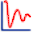Join one of our upcoming courses taking place around the world! Explore now

# Convergence Plot

The Convergence Plotoption in the Statistics menu, allows you to view the progress of a Probabilistic Analysis, to determine whether or not the analysis results are converging to constant values, and the approximate number of samples at which convergence occurs. This can help to determine:

• If you have used enough samples for the Probabilistic Analysis, or
• If you can use fewer samples, and still obtain valid results.

To create a Convergence Plot:

1. Select Convergence Plotfrom the toolbar or the Statistics menu.
2. In the Convergence Plot dialog:
1. Select the Data to Plot for which you would like to view results.
2. Select the Iteration Data that you would like to plot (see below for details).
3. Optionally select the Logarithmic X-Axis checkbox to plot the x-axis on a base-10 logarithmic plot
4. Optionally select the Show Bounding Curves checkbox to plot the minimum and maximum bounds of the Iteration Data.
5. Select the Plot button to generate the plot.

Once a plot is generated, there are several options available, if you right-click the mouse on the plot. See below for details.

## Data to Plot

The following analysis results can be plotted on a Convergence Plot:

• Factor of Safety
• Buckling Parameter
• Pillar Volume
• Pillar Weight
• Stope Area
• Shear Resistance
• Any random variables

## Iteration Data

The following value types can be plotted:

• Mean
• Variance

The mean and variance plots are obtained by recalculating the mean and variance of the results, after EACH sample iteration of the Probabilistic Analysis.

All of these plots should eventually converge to constant values, as the Number of Samples increases.

• If the plots do NOT converge to constant values, then you should INCREASE the Number of Samples used for the Probabilistic Analysis.
• Conversely, if the plots converge quickly, then you might DECREASE the Number of Samples, if desired, and still obtain similar final results, with less computation.

## Right-Click Options

Several options are available in the right-click menu for Convergence Plots, including: